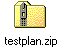The attached testplan is an example of two techniques:

1. Searching for an instrument setting value to give a particular measured value (i.e. what value of input power gives a particular output power) and then using the value found in a second search (i.e. searching for and finding an input power that gives a particular output power and then using that value of input power when doing a search for another value).

2. Doing searches and then using the values from the searches to make measurements over a range of another instrument setting values (i.e. searching for an input power that gives a particular output power and doing this for a range of frequencies).https://roos.com/docs/RBEH-4MERCE
ROOS INSTRUMENTS CONFIDENTIAL AND PROPRIETARY